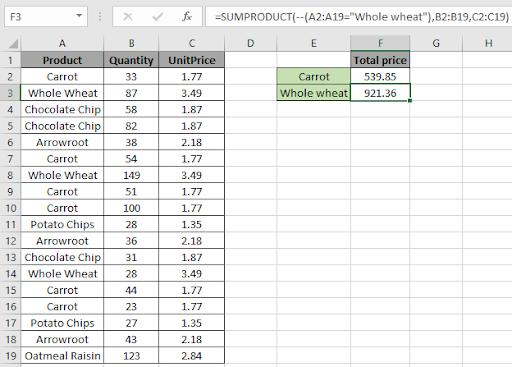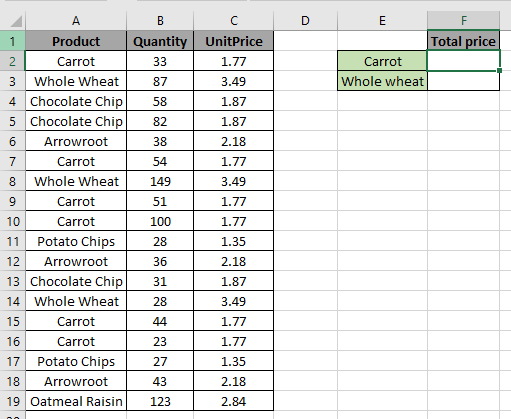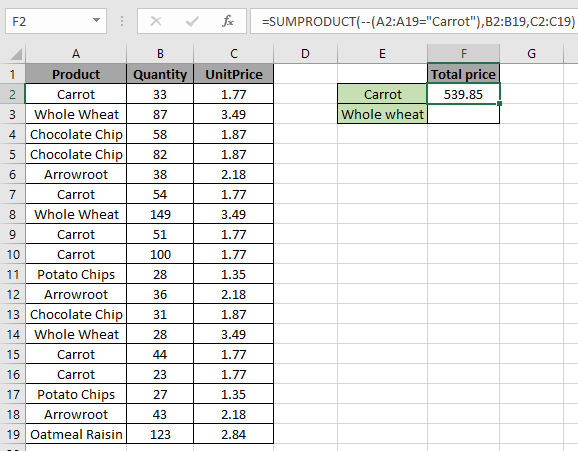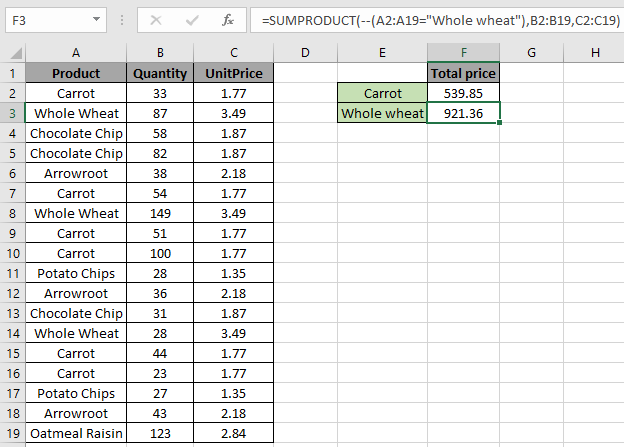# SUMPRODUCT with IF logicIn this article, we will learn about how to use IF logic with SUMPRODUCT function in Excel.

In simple words, SUMPRODUCT function take the product of the corresponding values and returns the sum of all. SUMPRODUCT function also use -- (operator) to meet certain criteria.

Generic Formula:

=SUMPRODUCT(--( array1=”condition”) , array2 , array3)

array1 : where criteria meet

array2 & array3 : these arrays will gets the product of corresponding values and returns their sum.

Example:

All of these might be confusing to understand. So, let's test this formula via running it on the example shown below.Here the problem is we need to find the total price for the product “Carrot”.
So here we use the formula to get the total price for Carrot

=SUMPRODUCT(--(A2:A19="Carrot"), B2:B19, C2:C19)

A2:A19 :  checks the range A2:A19 for the text “Carrot” and returns True if finds “Carrot” or else False

-- : the operator decodes True as 1 and False as 0.

B2:B19 : Quantity (range) to be multiplied with Unit Price.

C2:C19 : Unit price (range) to be multiplied with Quantity.Product Quantity UnitPrice Price 1 33 1.77 58.41 0 87 3.49 0 0 58 1.87 0 0 82 1.87 0 0 38 2.18 0 1 54 1.77 95.58 0 149 3.49 0 1 51 1.77 90.27 1 100 1.77 177 0 28 1.35 0 0 36 2.18 0 0 31 1.87 0 0 28 3.49 0 1 44 1.77 77.88 1 23 1.77 40.71 0 27 1.35 0 0 43 2.18 0 0 123 2.84 0 539.85

As you have understood from the above snapshot and  table, that SUMPRODUCT function multiplies corresponding values and returns the Sum as outcome.

1’s & 0’s in the left most column is the IF logic used in SUMPRODUCT function.

So here we use the formula for total price for Whole wheat

=SUMPRODUCT(--(A2:A19="Whole wheat"),B2:B19,C2:C19)

A2:A19: checks the range A2:A19 for the text “Whole wheat” and returns True if finds “Carrot” or else False
-- : the operator decode True as 1 and False as 0.
B2:B19 : Quantity (range) to be multiplied with Unit Price.
C2:C19 : Unit price (range) to be multiplied with Quantity.Product Quantity UnitPrice Price 0 33 1.77 0 1 87 3.49 303.63 0 58 1.87 0 0 82 1.87 0 0 38 2.18 0 0 54 1.77 0 1 149 3.49 520.01 0 51 1.77 0 0 100 1.77 0 0 28 1.35 0 0 36 2.18 0 0 31 1.87 0 1 28 3.49 97.72 0 44 1.77 0 0 23 1.77 0 0 27 1.35 0 0 43 2.18 0 0 123 2.84 0 921.36

As you have understood from the above snapshot and  table, that SUMPRODUCT function multiplies corresponding values and returns the Sum.

1’s & 0’s in the left most column is the IF logic used with SUMPRODUCT function.

Hope this article about how to SUMPRODUCT with IF logic in Excel is explanatory. Find more articles on SUMIF functions here. If you liked our blogs, share it with your fristarts on Facebook. And also you can follow us on Twitter and Facebook. We would love to hear from you, do let us know how we can improve, complement or innovate our work and make it better for you. Write to us at info@exceltip.com.

Related Articles

How to use the SUMPRODUCT function in Excel: Returns the SUM after multiplication of values in multiple arrays in excel.

SUM if date is between : Returns the SUM of values between given dates or period in excel.

Sum if date is greater than given date: Returns the SUM of values after the given date or period in excel.

2 Ways to Sum by Month in Excel: Returns the SUM of values within a given specific month in excel.

How to Sum Multiple Columns with Condition: Returns the SUM of values across multiple columns having condition in excel

How to use wildcards in excel : Count cells matching phrases using the wildcards in excel

Popular Articles

50 Excel Shortcut to Increase Your Productivity : Get faster at your task. These 50 shortcuts will make you work even faster on Excel.

How to use the VLOOKUP Function in Excel : This is one of the most used and popular functions of excel that is used to lookup value from different ranges and sheets.

How to use the COUNTIF function in Excel : Count values with conditions using this amazing function. You don't need to filter your data to count specific values. Countif function is essential to prepare your dashboard.

How to Use SUMIF Function in Excel : This is another dashboard essential function. This helps you sum up values on specific conditions.

1.•